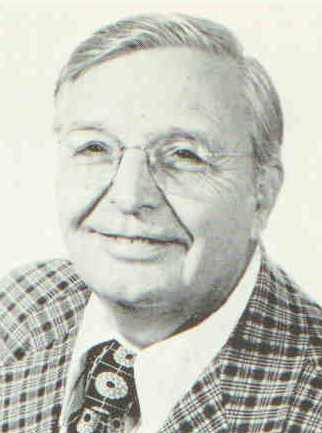Enter the content which will be displayed in sticky bar

# MemberDr. Robert L. Carroll
local time: 2021-06-15 06:46 (-04:00 DST)
Dr. Robert L. Carroll (Abstracts)
Titles
Abstracts Details
• [Updated 9 years ago]

It is shown that the field of physics is limited in its development by the perpetuation of erroneous concepts from the past. This fact applies to the concept of Euclidean space, to Newton?s laws of motion, and to the work of Einstein. Corrections are made, and it is shown that space has no meaning aside from energy. The present measure of universal energy is low by a factor equal to the number of positrons and electrons in existence.

• [Updated 9 years ago]

It is shown that Newton's second law is valid only as applied to a particle in which a collapsing field releases energy that goes into a mass increase. The third law applies to mechanics but requires that contact forces are conservative. The kinetic energy is increased at the expense of the internal energy.
Analysis indicates that energies are not additive in quantum theory or in electromagnetic theory. Toroidal matter is introduced and a study of electron clusters is made. The decay energy of the cluster is shown to be in excess of the ballistic energy of formation. This fact is in accord with reported experimental results. The implication is that contraction energy is involved. No explanation of the sources of the added energy is attempted

• The Photon (1996) [Updated 1 decade ago]

This article is written to show that the photon is a wave motion limited to one wavelength in the longitudinal direction and a transverse circumference of one wavelength. This gives it the form of a cylinder with no indication of any spread with distance of travel. Thus it has the characteristics of both wave and particle. The ?either-or? controversy of wave or particle is avoided in this way. There is no indication that a medium is required for its propagation.

• The Law of Motion (1994) [Updated 1 decade ago]

Published by Carroll Research Institute

• [Updated 1 decade ago]

• The Black Hole (1993) [Updated 3 years ago]

The action of the atomic clock is interpreted to show that time is an invariant form. The proper interpretation requires an internal reduction in the energy of matter for any velocity imposed. The law of the conservative field is applied to correct the Einstein equation for free fall. It is found that the action of contact forces is conservative as long as no radiation occurs. These facts are applied to show that the Black Hole as envisioned in the study of Cosmology has no existence in the real universe.

• The Mass-Space Principle (1993) [Updated 1 decade ago]

The fallacy of treating space and matter as separate entities is demonstrated. The Lorentz transformations are shown self-consistent, but physically impossible. The Space-Mass Principle is introduced to show that there is no background of space separate from matter which determines it.

• The Nature of Time (1992) [Updated 4 years ago]

The nature of the conservative field is used to show that the application of contact forces cannot change the total energy of an object. Mathematical analysis indicates that field forces are velocity-dependent. The concept that the reactive force does not work is shown in error. Analysis shows that the relativity time transformation has no validity in the sense of a time dilation, but indicates a reduction in the internal energy of an accelerated object, and hence the slowing of periodic processes.

• [Updated 1 decade ago]

By applying quantum theory to fluid mechanics, it is shown why superconductivity was considered a low temperature phenomenon, but that under certain circumstances electron pairing and high currents in filaments will occur. In particular, it is found that bismuth at room temperatures cannot be far from superconductivity.

• The Toroidal Electron (1991) [Updated 1 decade ago]

Reasons are given for introducing half integers as separation constants in solving the general wave equation associated with matter. The results are interpreted as a description of two types of elementary particles, electrons and positrons, each with two types of spin, with an internal circulation velocity equal to the velocity of light when the particle is at rest, but in general dependent on the translational velocity. Energy and momentum relations are also derived.

• [Updated 1 decade ago]

The simplest concept of physics is that of the equality of the forces of action and reaction. If two ojects interact by contact, an equality of magnitudes between the applied and inertial forces is required. The applied force does work upon the object with which it is in contact. The inertial force, it is thought, does no work. The problem is how to define which force is the one applied and which object exerts the inertial force. In the absence of an external reference frame, we can say the second object was at rest when it was struck by the first. Therefore the inertial force was generated by the second object. The viewpoint that the second object was struck by the first is just as valid, but requires that the inertial force should be generated by the first object. Since it is impossible to define which force is inertial, and which is not, we abandon the concept that the inertial force does no work. The analysis then leads to the internal energy of matter.

• The Nature of Space (1990) [Updated 1 decade ago]

The paper shows that the concept of space is bound to matter and its energy, in particular gravitational, electromagnetic, and rotational energy. It follows that the universe must be finite and cannot be steadily expanding.